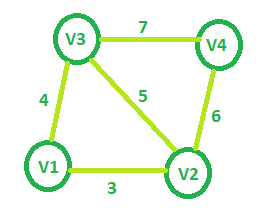# Minimum spanning tree cost of given Graphs

Given an undirected graph of V nodes (V > 2) named V1, V2, V3, …, Vn. Two nodes Vi and Vj are connected to each other if and only if 0 < | i – j | ≤ 2. Each edge between any vertex pair (Vi, Vj) is assigned a weight i + j. The task is to find the cost of the minimum spanning tree of such graph with V nodes.

Examples:

Input: V = 4Output: 13

Input: V = 5
Output: 21

## Recommended: Please try your approach on {IDE} first, before moving on to the solution.

Approach: Starting with a graph with minimum nodes (i.e. 3 nodes), the cost of the minimum spanning tree will be 7. Now for every node i starting from the fourth node which can be added to this graph, ith node can only be connected to (i – 1)th and (i – 2)th node and the minimum spanning tree will only include the node with the minimum weight so the newly added edge will have the weight i + (i – 2).

So addition of fourth node will increase the overall weight as 7 + (4 + 2) = 13
Similarly adding fifth node, weight = 13 + (5 + 3) = 21

For nth node, weight = weight + (n + (n – 2)).

This can be generalized as weight = V2 – V + 1 where V is the total nodes in the graph.

Below is the implementation of the above approach:

## C++

 `// C++ implementation of the approach ` `#include ` `using` `namespace` `std; ` ` `  `// Function that returns the minimum cost ` `// of the spanning tree for the required graph ` `int` `getMinCost(``int` `Vertices) ` `{ ` `    ``int` `cost = 0; ` ` `  `    ``// Calculating cost of MST ` `    ``cost = (Vertices * Vertices) - Vertices + 1; ` ` `  `    ``return` `cost; ` `} ` ` `  `// Driver code ` `int` `main() ` `{ ` `    ``int` `V = 5; ` `    ``cout << getMinCost(V); ` ` `  `    ``return` `0; ` `} `

## Java

 `// Java implementation of the approach  ` `class` `GfG  ` `{  ` ` `  `// Function that returns the minimum cost  ` `// of the spanning tree for the required graph  ` `static` `int` `getMinCost(``int` `Vertices)  ` `{  ` `    ``int` `cost = ``0``;  ` ` `  `    ``// Calculating cost of MST  ` `    ``cost = (Vertices * Vertices) - Vertices + ``1``;  ` ` `  `    ``return` `cost;  ` `}  ` ` `  `// Driver code  ` `public` `static` `void` `main(String[] args)  ` `{  ` `    ``int` `V = ``5``;  ` `    ``System.out.println(getMinCost(V));  ` `} ` `}  ` ` `  `// This code is contributed by  ` `// Prerna Saini. `

## C#

 `// C# implementation of the above approach  ` `using` `System; ` ` `  `class` `GfG  ` `{  ` ` `  `    ``// Function that returns the minimum cost  ` `    ``// of the spanning tree for the required graph  ` `    ``static` `int` `getMinCost(``int` `Vertices)  ` `    ``{  ` `        ``int` `cost = 0;  ` `     `  `        ``// Calculating cost of MST  ` `        ``cost = (Vertices * Vertices) - Vertices + 1;  ` `     `  `        ``return` `cost;  ` `    ``}  ` `     `  `    ``// Driver code  ` `    ``public` `static` `void` `Main()  ` `    ``{  ` `        ``int` `V = 5;  ` `        ``Console.WriteLine(getMinCost(V));  ` `    ``} ` `}  ` ` `  `// This code is contributed by Ryuga `

## Python3

 `# python3 implementation of the approach ` `  `  `# Function that returns the minimum cost ` `# of the spanning tree for the required graph ` `def` `getMinCost( Vertices): ` `    ``cost ``=` `0` `  `  `    ``# Calculating cost of MST ` `    ``cost ``=` `(Vertices ``*` `Vertices) ``-` `Vertices ``+` `1` `  `  `    ``return` `cost ` `  `  `# Driver code ` `if` `__name__ ``=``=` `"__main__"``: ` ` `  `    ``V ``=` `5` `    ``print` `(getMinCost(V)) ` ` `

## PHP

 ` `

Output:

```21
```

Attention reader! Don’t stop learning now. Get hold of all the important DSA concepts with the DSA Self Paced Course at a student-friendly price and become industry ready.

My Personal Notes arrow_drop_upCheck out this Author's contributed articles.

If you like GeeksforGeeks and would like to contribute, you can also write an article using contribute.geeksforgeeks.org or mail your article to contribute@geeksforgeeks.org. See your article appearing on the GeeksforGeeks main page and help other Geeks.

Please Improve this article if you find anything incorrect by clicking on the "Improve Article" button below.

Article Tags :
Practice Tags :

1

Please write to us at contribute@geeksforgeeks.org to report any issue with the above content.